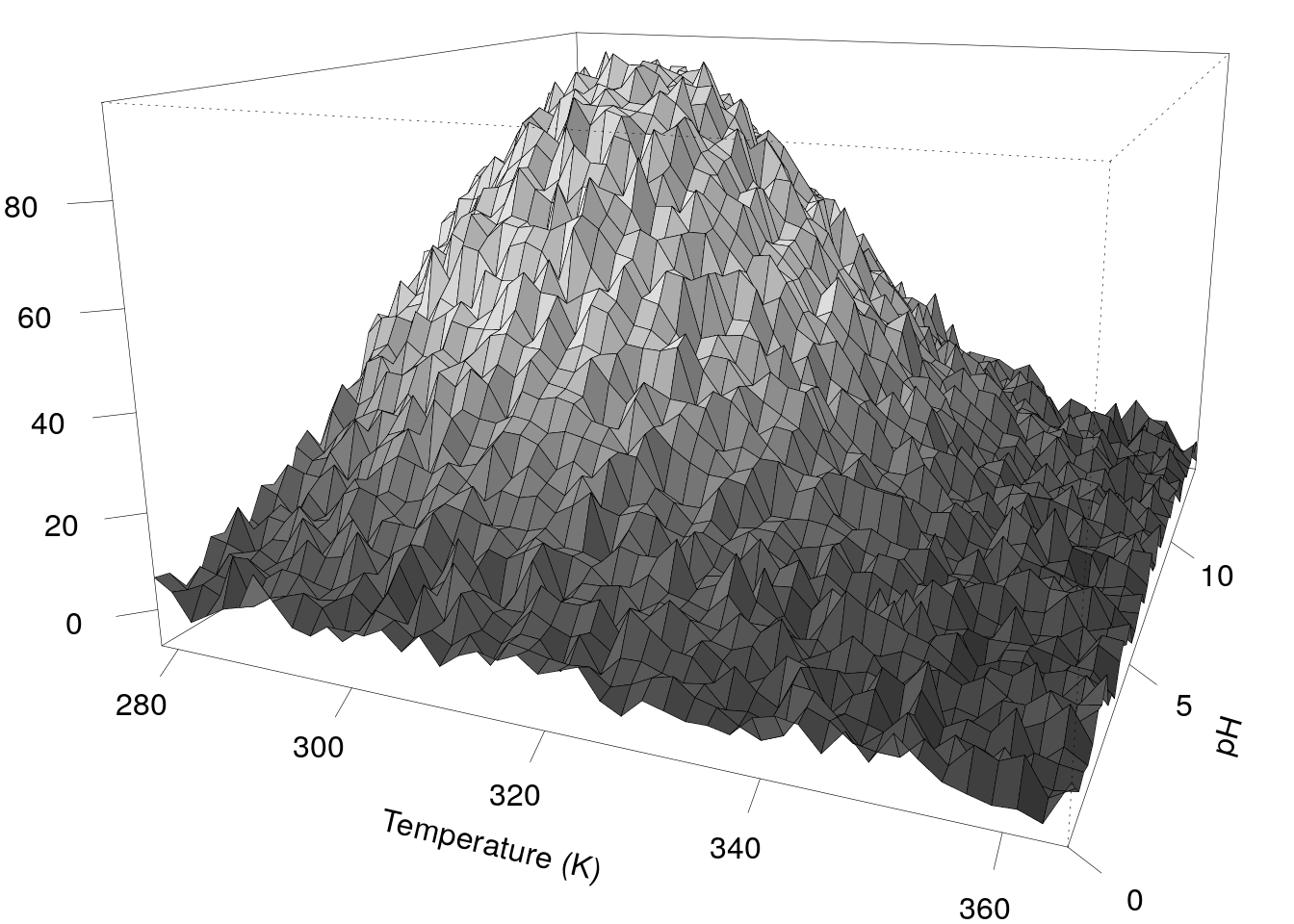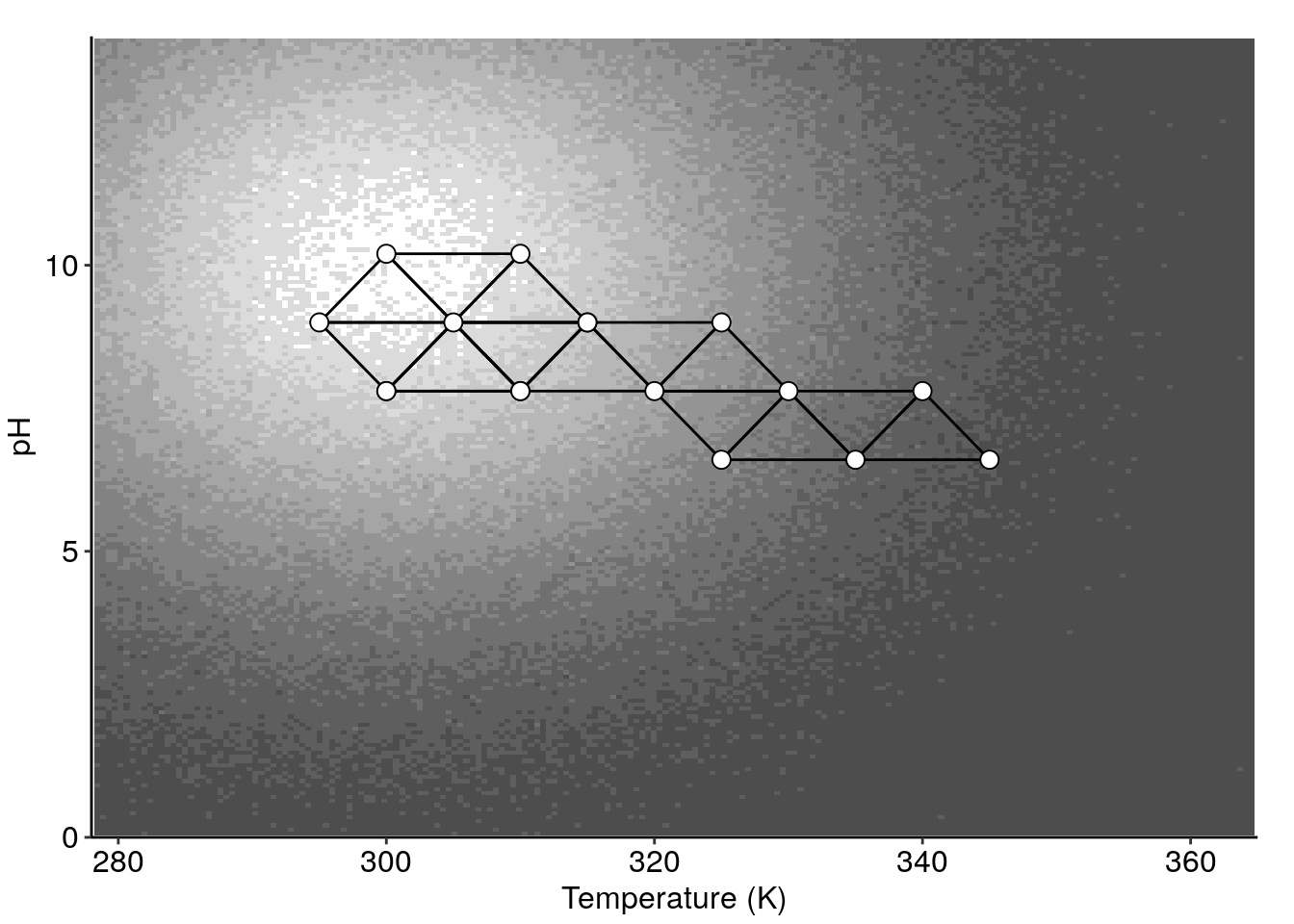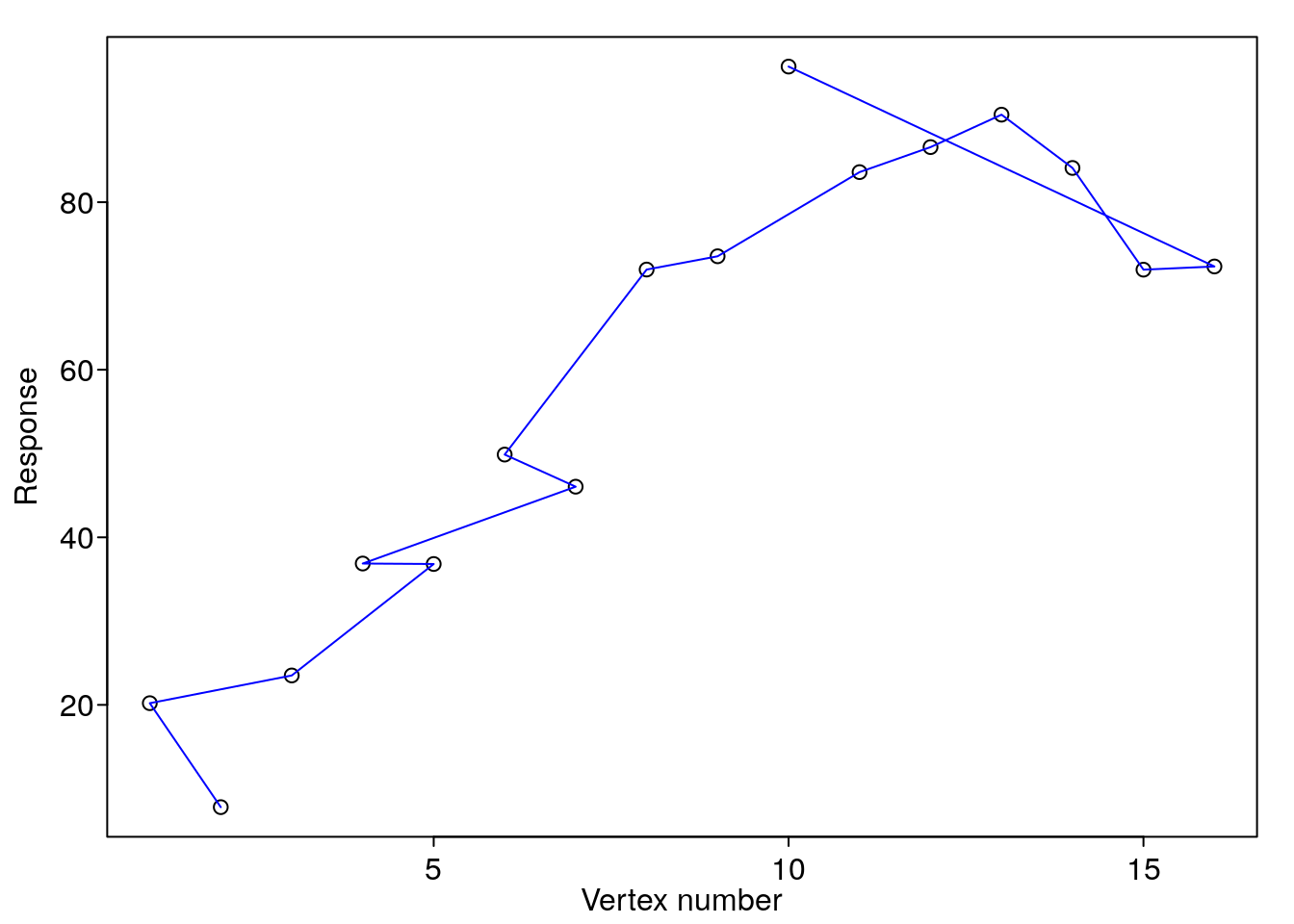# labsimplex package

#### 2020-06-03

The R package `labsimplex` implements the simplex optimization algorithms firstly proposed by Spendley, Hext, and Himsworth (1962) and later modified by Nelder and Mead (1965) for the improvement of laboratory and manufacturing processes. This package provides tools for visualizing the coordinates of the experimental variables and the evolution in the response as a function of the number of experiments. The `labsimplex` package is not intended for linear programming applications. For this purpose, we encourage you to check the `optimsimplex` package also available at CRAN (Bihorel and Baudin 2018).

## Introduction

A simplex is a geometric element defined as the simpler polytope possible in an n-dimensional space. If the space has n dimensions, the simplexes there will have n+1 corners called vertexes. The simplexes in two and three-dimensional spaces are the well-known triangle and tetrahedron, respectively.

In the simplex optimization algorithms, the experimental variables are represented by the dimensions in the abstract space. Each vertex in the simplex represents an experiment and the coordinates of the vertex represent the values for the variables in that experimental setting. The experiments must be performed and a response must be assigned to each vertex. In the optimization process, one of the vertexes is discarded in favor of a new one that must be evaluated. In the first simplex, the vertex with the worst response is discarded. The second worst vertex in this simplex is discarded in the following simplex and the procedure is repeated until the optimum is reached or a response good enough is obtained. Discarding a vertex and generating a new one is known as a movement of the simplex.

The fixed step-size simplex algorithm introduced by Spendley, Hext, and Himsworth (1962) is based on the idea that getting away from the zone that yields the worst results will provide a close up to the optimal zone.

## Installation

The last released version of the package `labsimplex` can be installed from CRAN by running:

``install.packages("labsimplex")``

The development version of labsimplex can be installed from GitHub using `install_github()` function from `devtools` package (Wickham, Hester, and Chang 2019):

``devtools::install_github("Crparedes/labsimplex", build_vignettes = TRUE)``

## Using the package

The first step is to define the quantitative response that is intended to improve and the variables to optimize. The initial values for those variables and a convenient step-size for each variable must be defined. One of the advantages of the simplex algorithm over many Design of Experiments strategies is that the inclusion of more variables to study does not significantly increase the number of experiments to perform. This fact usually makes unnecessary the process of screening the variables The optimization using `labsimplex` package involves the following steps that will be further discussed in the next sections:

1. Generate the initial simplex.
2. Export the simplex to an external file to ensure the information will not be lost if the R session is restarted.
3. Perform the experiments described by the vertexes (or vertex) and assing response values.
4. Import the file created in the second step.
5. Generate the new vertex to be evaluated.
6. Repeat steps 2 to 5 until the desired response is obtained

If the experiments do not take long, the response may be obtained quickly and the process can be optimized in a single R session. In this case, the second and fourth steps that include exporting and importing information may be unnecessary. The simplex coordinates may be plotted in any stage of the process to have an idea of the path of the simplex in it’s way to the optimum region.

## A worked example: Optimizing the yield of a chemical reaction

The package includes the functions `exampleSurfaceR2()`, `exampleSurfaceR2.2pks()` and `exampleSurfaceR3()` that model the yield of hypothetical chemical reactions that are affected by pH, temperature and concentration (the latter only for `exampleSurfaceR3()`). Those functions produce response surfaces that can be used to simulate the obtention of a result when the reactions are performed under the conditions given by the simplex algorithm. The main functions of the package are illustrated in this section using the reaction modeled by `exampleSurfaceR2()`. The shape and contours of this function are shown in Figure 1.

``````prspctv(surface = exampleSurfaceR2, par = list(mar = c(0.5, 0.6, 0, 0)), phi = 30, theta = 30,
ltheta = -120, expand = 0.6, xlab = 'Temperature (K)', ylab = 'pH', zlab = 'Yield (%)')
(cont.surf <- cntr(surface = exampleSurfaceR2, length = 200))``````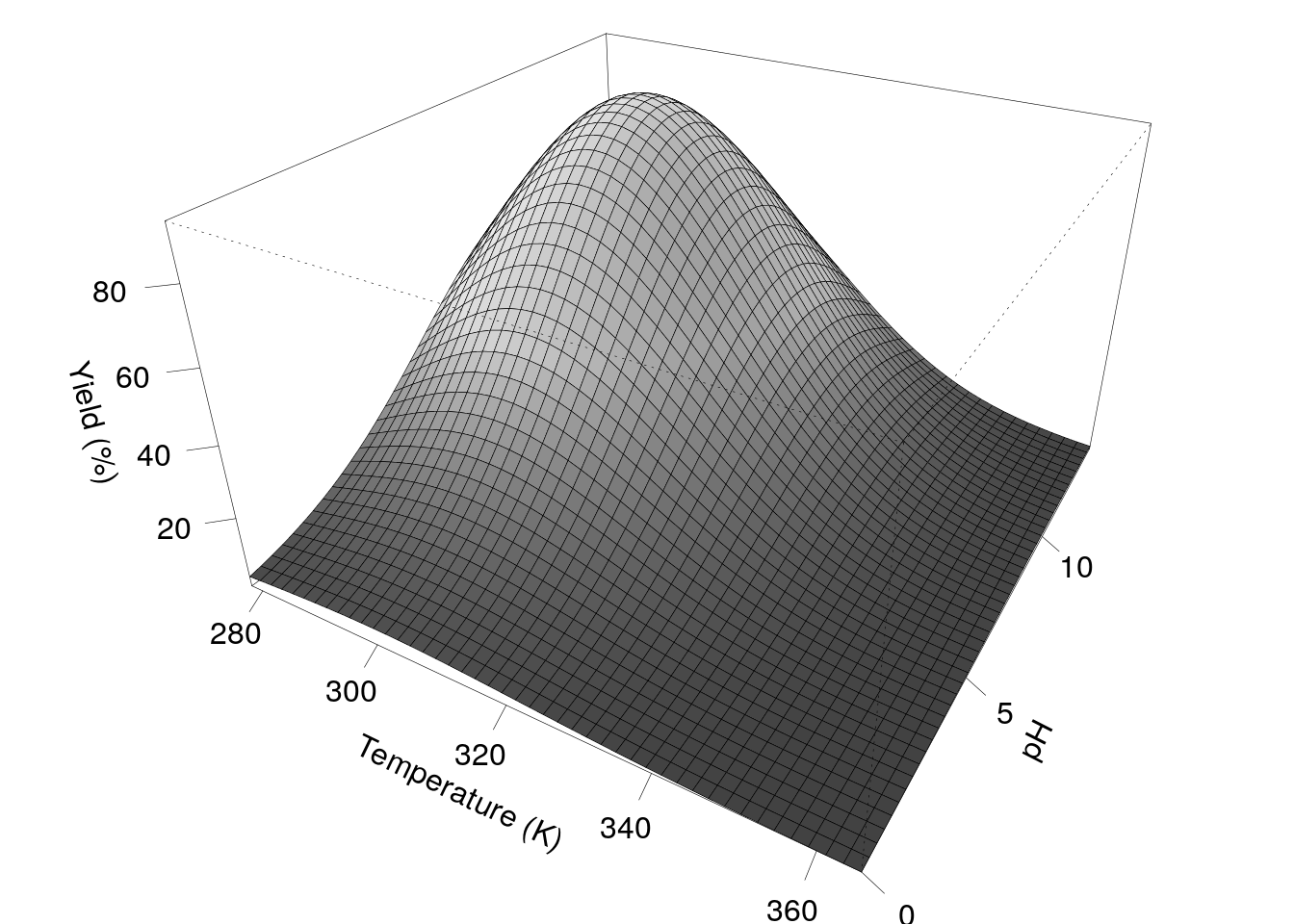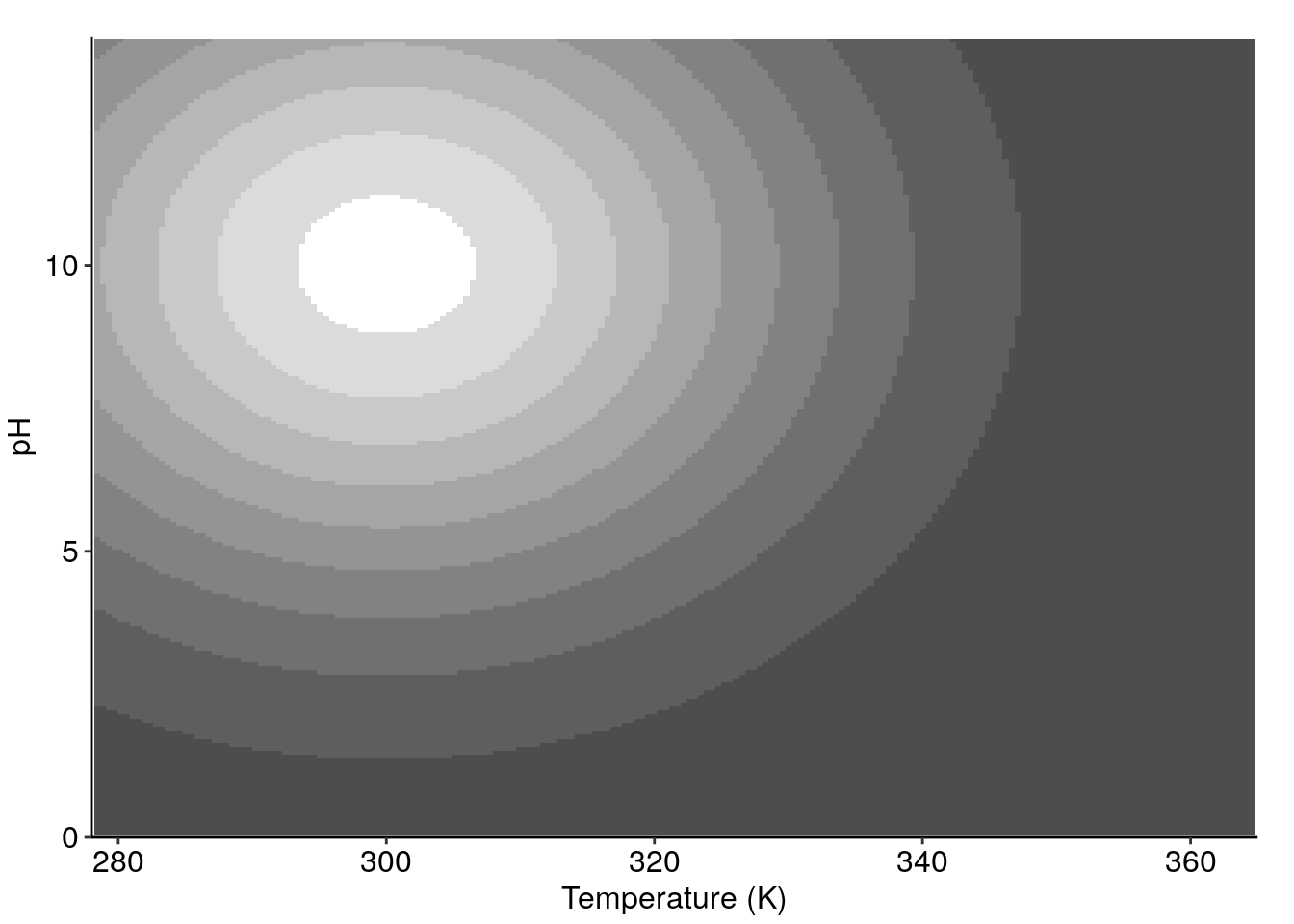Response surface `exampleSurfaceR2()` in 3D perspective (left) and contour plot (right).

### Generating the initial simplex

The function `labsimplex()` creates a new simplex object. The only not optional parameter is `n` which relates to the dimensionality of the simplex. In this example only temperature and pH are considered, then `n = 2`. If just `n` is provided, the function generates a regular simplex centered at the origin. That simplex must be transformed into the real coordinates space by providing a spatial reference (the `centroid` or the `start` vertex) and a size reference (`stepsize`) for each coordinate. Variable names may be specified as a character vector in the `var.name` parameter. To create a simplex centered at pH 7 and a temperature of 340 K with a step-size of 1.2 and 10 K for pH and temperature, respectively, use:

``````simplexR2 <- labsimplex(n = 2, centroid = c(7, 340), stepsize = c(1.2, 10),
var.name = c('pH', 'Temperature'))
print(simplexR2)
#> Current simplex:
#>            pH Temperature . Response Label Nature
#> Vertex.1: 7.8         340 |       NA    NA      S
#> Vertex.2: 6.6         345 |       NA    NA      S
#> Vertex.3: 6.6         335 |       NA    NA      S
#>
#> Conventions:
#>     Labels:                    Nature:
#>        W: Worst or Wastebasket    S:  Starting
#>        N: Next to the worst       R:  Reflected
#>        B: Best                    E:  Expanded
#>                                   Cr: Contraction on the reflection side
#>        D: Disregarded             Cw: Contraction on the worst side
#>
#>       Use print(..., conventions = FALSE) to disable conventions printing.``````

When calling `print(simplex)` the output consists of three main sections. The first one contains the information of the vertexesin the current simplex. The second section (not shown here) has information on historical vertexes that were previously evaluated and discarded. The third section shows the conventions used in the tables. The conventions printing may be disabled using `print(..., conventions = FALSE)`. The information of each vertex has an identifier (Vertex.##), the coordinates for each of its n variables, the response, the label (best, next to the worst, worst or wastebasket or disregarded vertex), and its nature (vertex of the initial simplex or generated as a reflection, expansion or contraction of the previous simplex).

##### Explicit definition of the initial simplex

It is possible to manually define the coordinates of the vertexes in the initial simplex. Those coordinates are provided in the `usrdef` parameter as an (n+1 x n) matrix, with each row representing a vertex and each column, a variable. When defining the vertex coordinates, other parameters regarding spatial or size references are incompatible.

``````coords <- rbind(c(7.1, 325), c(6.5, 350), c(6.5, 300))
simplexR2Manual <- labsimplex(n = 2, usrdef = coords, var.name = c('pH', 'Temperature'))
#> Provided points define a simplex:
print(simplexR2Manual, conventions = FALSE)
#> Current simplex:
#>            pH Temperature . Response Label Nature
#> Vertex.1: 7.1         325 |       NA    NA      S
#> Vertex.2: 6.5         350 |       NA    NA      S
#> Vertex.3: 6.5         300 |       NA    NA      S``````

Setting the simplex coordinates explicitly will fail if the vertexes are linearly dependent. This scenario is known as cohypoplanarity and produces a degenerate simplex that is not capable of freely explore the experimental space (Walters et al. 1991). The probability of defining a degenerate simplex grows up with the dimensionality of the space. If the simplex introduced by the user is correct, the function returns a message `Provided points define a simplex`.

###### Two-level fractional factorial desings in the generation of the initial simplex

It is common to perform a two-level fractional factorial design to screen the variables that affect a process. As mentioned in the introduction, this is often unnecessary in the simplex optimization strategies as long as the inclusion of more variables does not significantly increase the number of experiments to perform. However, in most cases, those experiments may be used to produce the initial vertex with the advantage that if the experiments have already been performed, the responses are already available. In some cases, low resolution two-level fractional factorial designs are capable of studying n variables in n+1 experiments and those experiments define a well behaved (i.e. non-degenerate) simplex. At this point, the algorithm is ready to propose a new vertex that may be closer to the optimum zone.

For example, suppose the use of a two-level fractional factorial design of resolution III was used to study a chemical reaction affected by pH, temperature, and concentration. The R package `FrF2` (Grömping 2014) creates and analyzes fractional factorial two-level designs and is used in this section to study the described system. The low and high levels for the pH, the temperature and the concentration will be 6.8 and 7.2, 330, and 350 K and 0.4 and 0.6 (arbitrary units) respectively.

``````if (!require(FrF2)) message('Please install FrF2 package')
set.seed(1)
(screening <- FrF2(resolution = 3,
factor.names = list(pH = c(6.8, 7.2), Temp = c(330, 350), Conc = c(0.4, 0.6))))
#>    pH Temp Conc
#> 1 6.8  330  0.6
#> 2 6.8  350  0.4
#> 3 7.2  350  0.6
#> 4 7.2  330  0.4
#> class=design, type= FrF2``````

The variable coordinates and the names can be passed to the labsimplex function after minor transformations:

``````simplexR3 <- labsimplex(n = 3, usrdef = matrix(as.numeric(as.matrix(screening)), ncol = 3),
var.name = dimnames(screening)[])
#> Provided points define a simplex:
print(simplexR3, conventions = FALSE)
#> Current simplex:
#>            pH Temp Conc . Response Label Nature
#> Vertex.1: 6.8  330  0.6 |       NA    NA      S
#> Vertex.2: 6.8  350  0.4 |       NA    NA      S
#> Vertex.3: 7.2  350  0.6 |       NA    NA      S
#> Vertex.4: 7.2  330  0.4 |       NA    NA      S``````

If the number of experiments is greater than the number of variables plus one, some points are not necessary and again we have the risk of choosing a degenerate simplex. The `labsimplex()` function checks if the simplex has been correctly defined.

### Modifying vertex coordinates

In some cases, it is very hard to set an exact particular value for a variable in an experimental setting. Most of the time the little variations will not play an important effect on the experiment outcome. However, if it is desired to take into account the differences between the algorithm-proposed vertex and the actual experiment performed, the `modifyVertex()` function allows changing as many coordinates as desired before generating a new vertex.

In the first simplex created, suppose we want to change the pH of the first vertex to 7.9 and the temperature of the second vertex to 342 K. The changes are given in a list containing numeric vectors of length n. The manes of the list elements refer to the vertexes that are to be modified and must contain the new coordinates in the respective position. Other positions have `NA` values meaning that no changes are required.

``````adjustVertex(simplex = simplexR2, newcoords = list(Vertex.1 = c(7.95, NA), Vertex.2 = c(NA, 342)),
overwrite = TRUE)
print(simplexR2, conventions = FALSE)
#> Current simplex:
#>             pH Temperature . Response Label Nature
#> Vertex.1: 7.95         340 |       NA    NA      S
#> Vertex.2: 6.60         342 |       NA    NA      S
#> Vertex.3: 6.60         335 |       NA    NA      S``````

Observe that the parameter `overwrite = TRUE` makes the output of the function to replace (overwrite) the object given in `simplex = simplexR2`. This enhances code readability by removing the requirement of explicitly redefining the `simplexR2` object.

### Graphical representation of the simplex

The simplex may be visualized in two and three-dimensional plots using `plot()` and `plotSimplex3D()` functions. The suitability of one function over the other depends on the simplex dimensionality. `plot()` requires a simplex with at least two variables while `plotSimplex3D()` requires the number of variables to be at least three. If the simplex dimensionality is higher than the required by the functions, the variables to plot may be selected and the graphical representation produced will vary according to the variables selected. In this case, some vertexes may not appear for some combinations of variables. If no variables are indicated, by default the functions will plot the first two or three variables, respectively.

When the optimization is running over one of the example surfaces provided in the package, the function `addSimplex2Surface()` allows the visualization of the simplex optimization path over the contour surface. Both approaches are shown in Figure 2 `simplexR2` object previously created. The latter approach will be used for the rest of the document.

``````plot(simplexR2)
(addSimplex2Surface(p = cont.surf, simplex = simplexR2))``````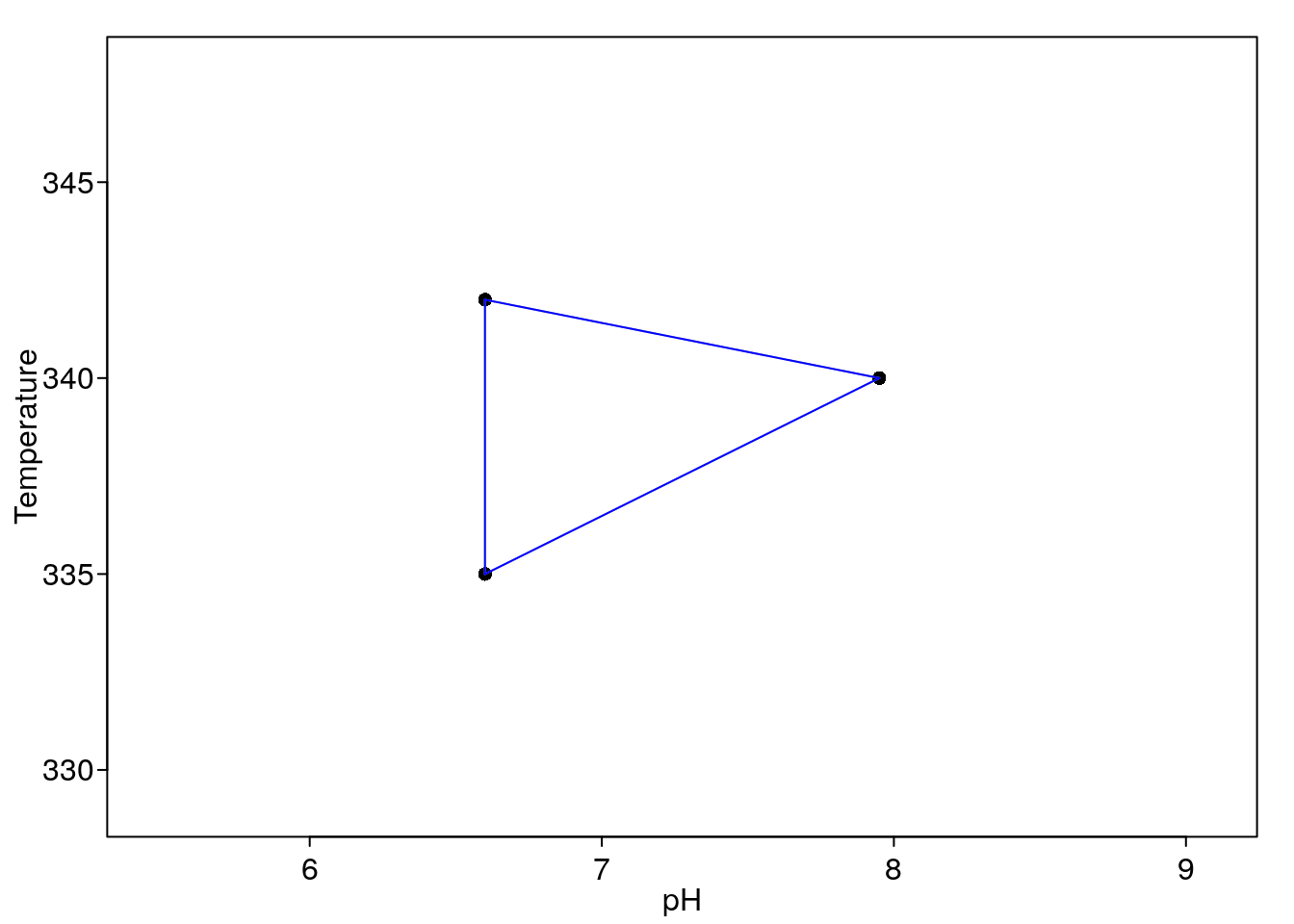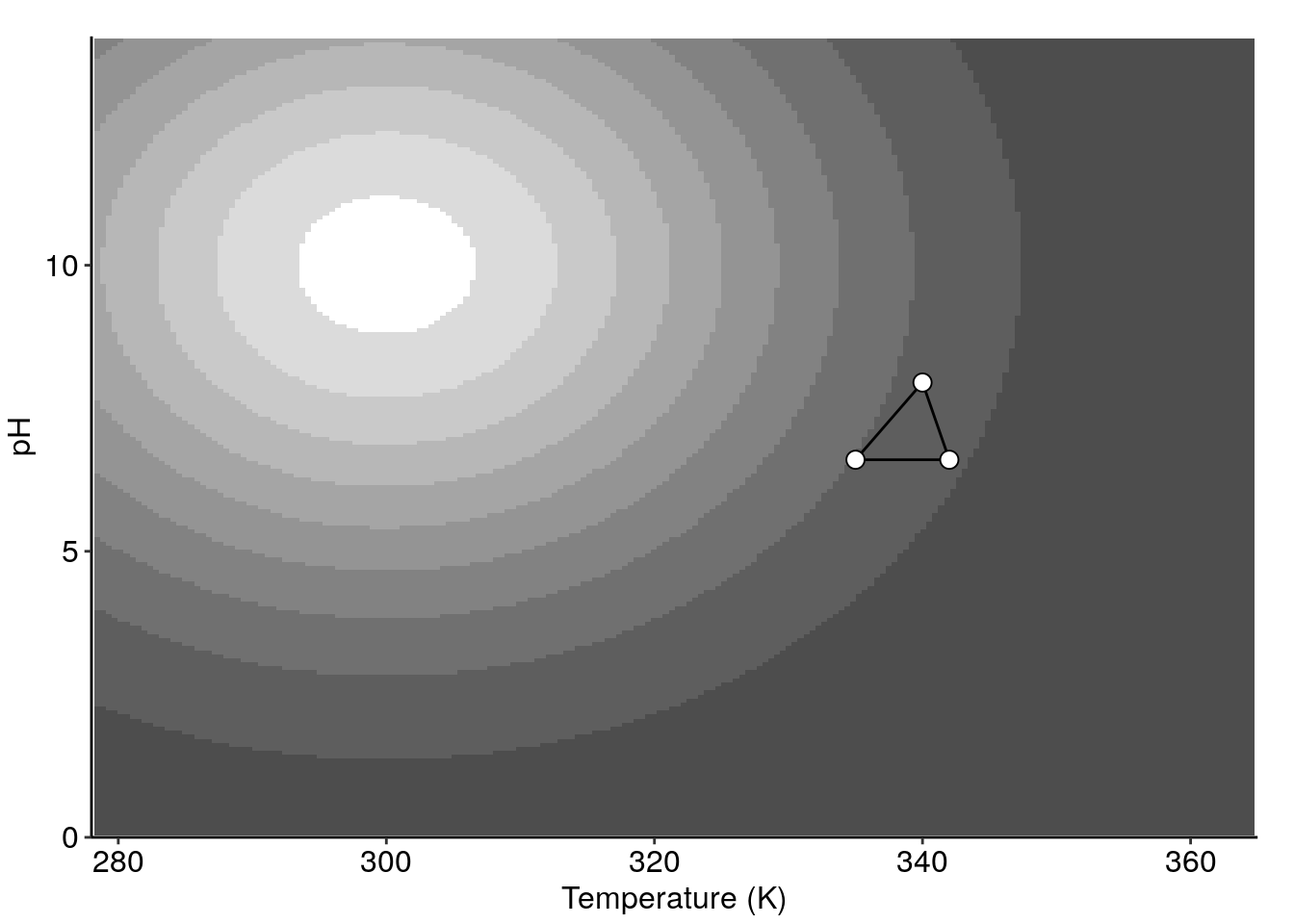Initial two-variables simplex isolated in the space (left) and over the response surface that describes the system (right).

### Generating new vertexes

To generate a new vertex, it is necessary to provide the responses of the previous vertexes, the optimization criteria and the algorithm to follow (fixed-size or variable-size). This means that it is necessary to perform the experiments proposed in the initial simplex. In this document, the responses are obtained according to the response surface shown in Figure 1. The function to use is `generateVertex()`.

``````(responses <- exampleSurfaceR2(x1 = simplexR2\$coords[, 2], x2 = simplexR2\$coords[, 1]))
#> Vertex.1 Vertex.2 Vertex.3
#> 16.73027 11.38726 19.52108
generateVertex(simplex = simplexR2, qflv = responses, crit = 'max',
algor = 'fixed', overwrite = TRUE)
#>          pH Temperature
#>        7.95      333.00
print(simplexR2, conventions = FALSE)
#> Current simplex:
#>             pH Temperature . Response Label Nature
#> Vertex.1: 7.95         340 | 16.73027     N      S
#> Vertex.3: 6.60         335 | 19.52108     B      S
#> Vertex.4: 7.95         333 |       NA  <NA>      R
#>
#> Historical Vertices:
#>            pH Temperature . Response Label Nature
#> Vertex.2: 6.6         342 | 11.38726     W      S``````

Observe that the parameter `overwrite = TRUE` can be also be used here to replace the old simplex by the new one without explicitly using the `<-` function. The experiment described by the new vertex must be performed and the process is repeated until the optimum is reached. The simplex first reflection and the simplex complete path to the optimum are shown in Figure 3.

``````(addSimplex2Surface(p = cont.surf, simplex = simplexR2))
simplexR2 <- exampleOptimization(surface = exampleSurfaceR2, simplex = simplexR2)
(addSimplex2Surface(p = cont.surf, simplex = simplexR2))``````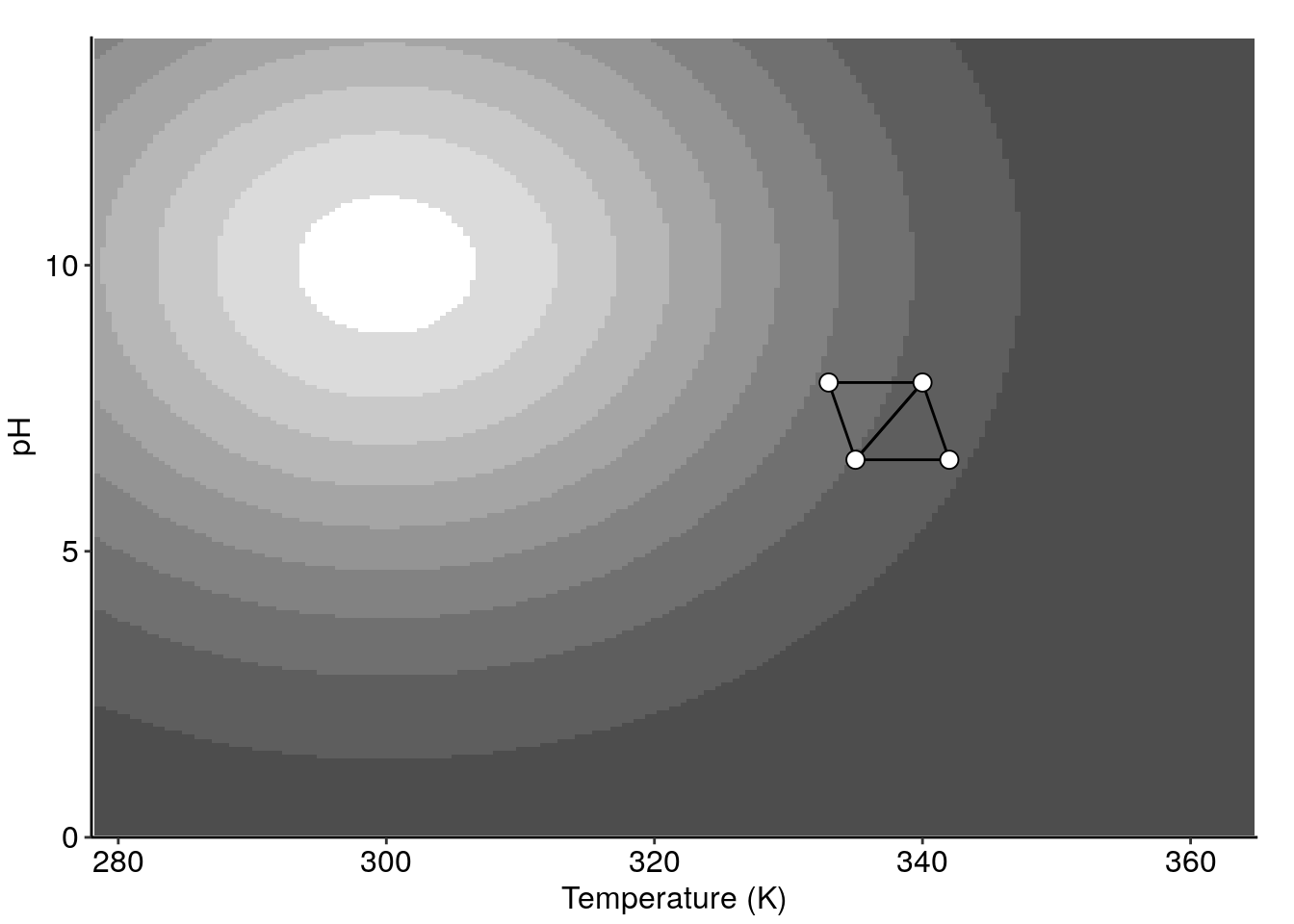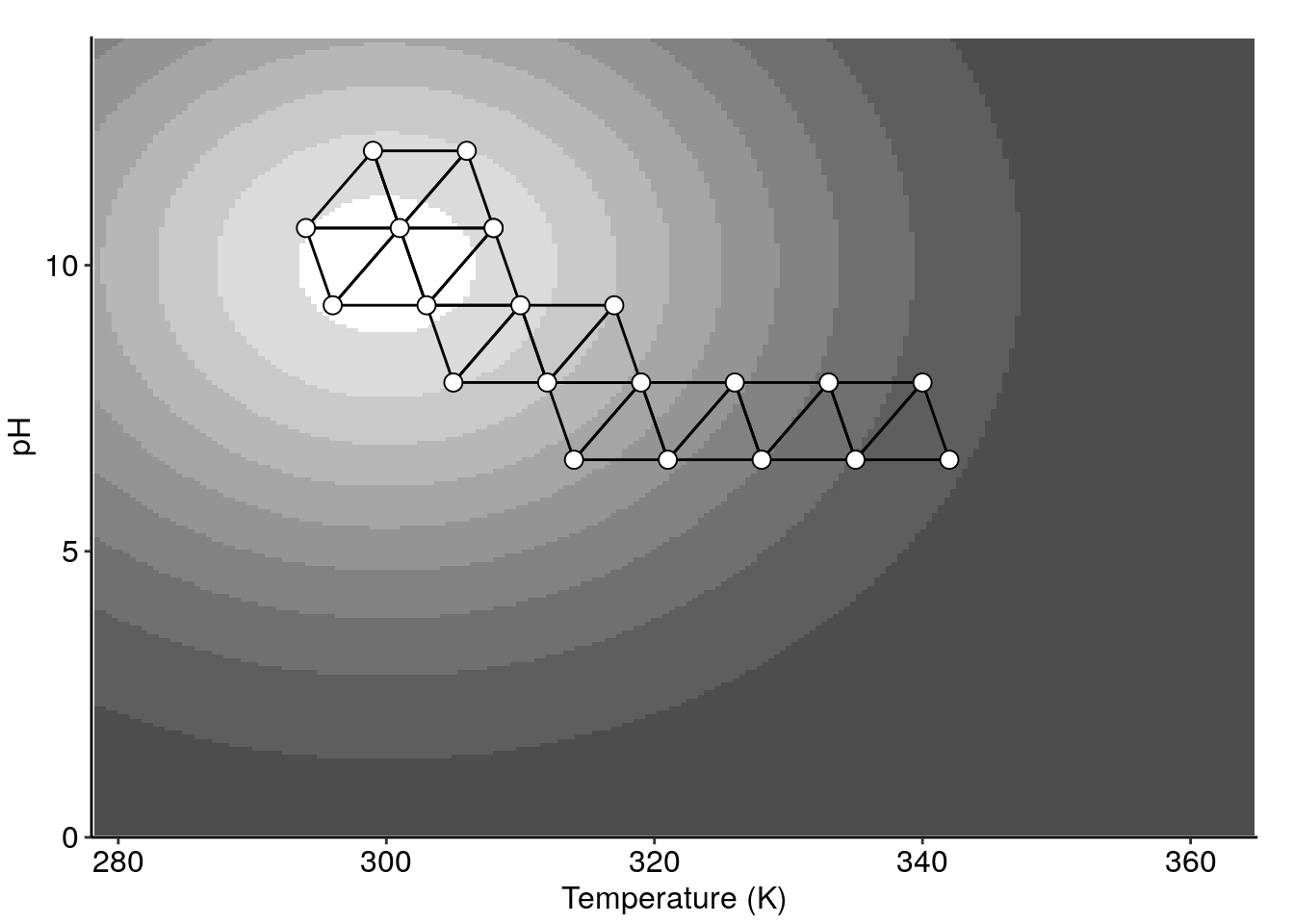First movement (left) and complete path (right) of a fixed step-size simplex optimazation over the response surface `exampleSurfaceR2()`.

When the algorithm reaches the optimum zone in the fixed-size simplex algorithm, the simplex starts to spin around the vertex that had the better response. Eventually the previously evaluated vertexes begin to being proposed again by the algorithm.

In the variable size algorithm, the simplex will contract into the optimal point indefinitely until variations became so small that it is practically impossible (or simply inconvenient from a practical point of view) to experimentally differentiate two vertices. The Figure 4 shows the complete path of the simplex to the optimal zone.

``````simplexR2Var <- exampleOptimization(surface = exampleSurfaceR2, algor = 'variable',
centroid = c(7, 340), stepsize = c(1.2, 10))
(addSimplex2Surface(p = cont.surf, simplex = simplexR2Var))
#> Warning: Removed 6 rows containing missing values (geom_segment).
#> Warning: Removed 3 rows containing missing values (geom_point).``````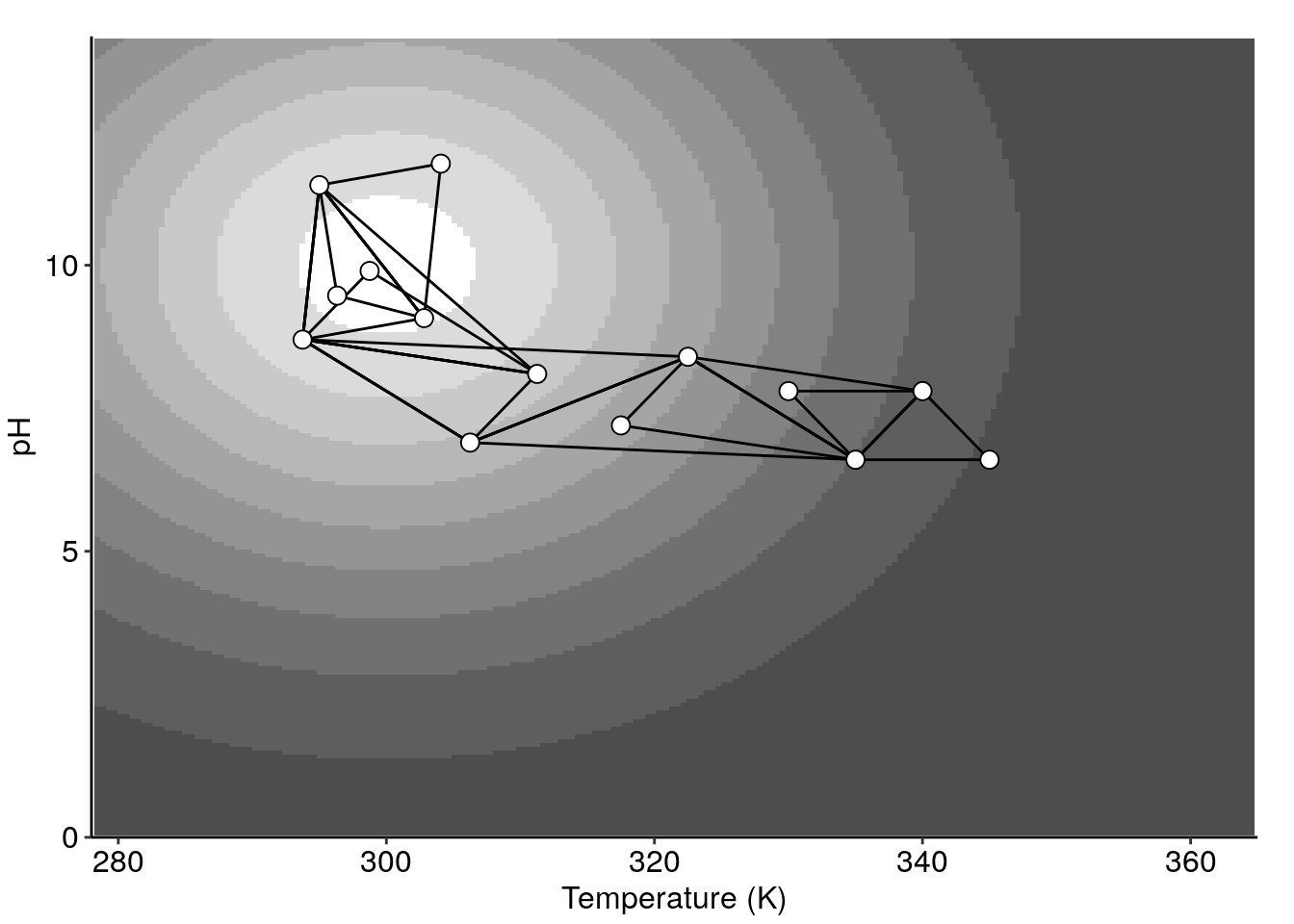Complete path of a variable step-size simplex optimazation over the response surface `exampleSurfaceR2()`.

The response value can be plotted against the vertex number using the function `plotSimplexResponse()`.

``````plotSimplexResponse(simplexR2)
plotSimplexResponse(simplexR2Var)``````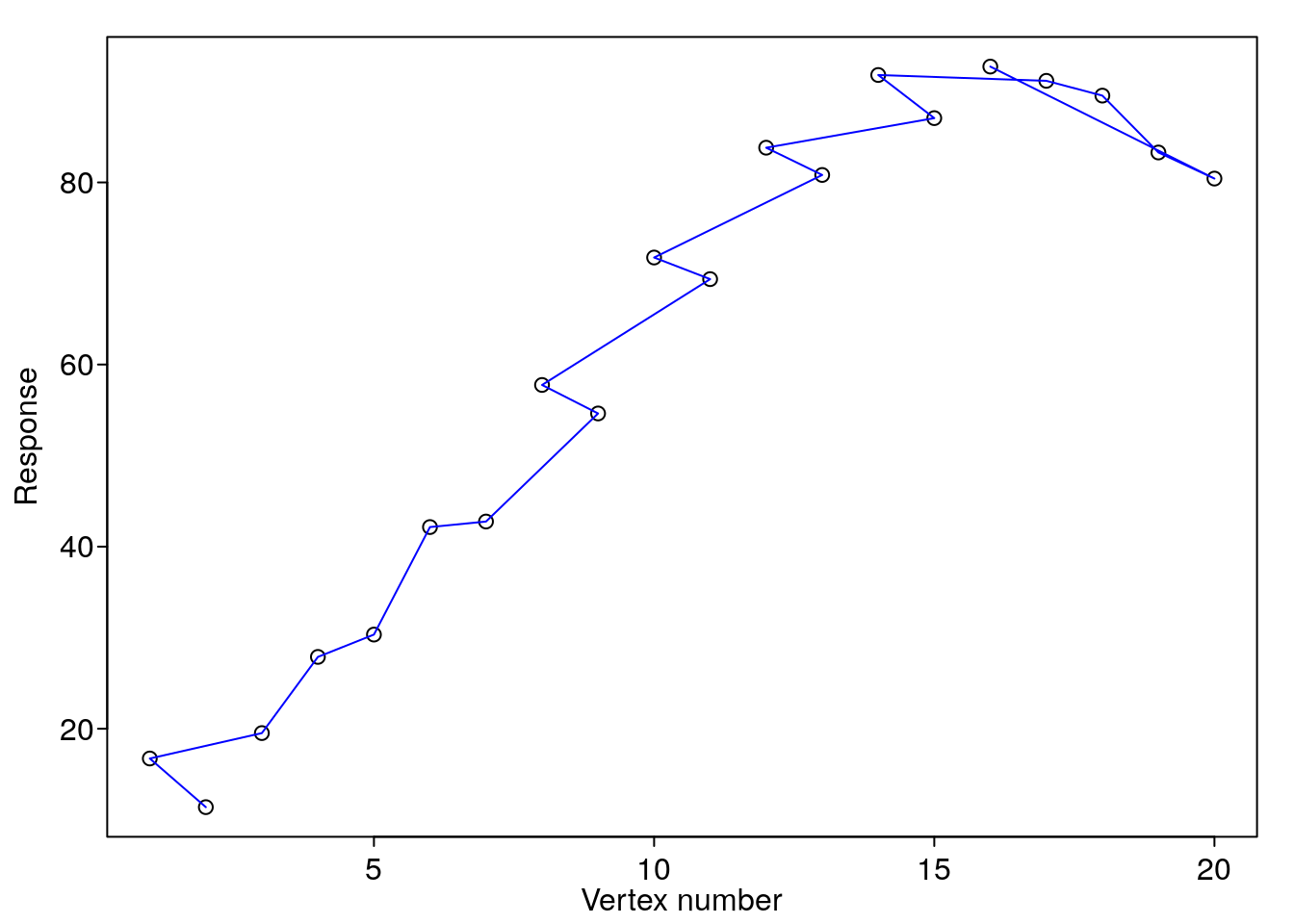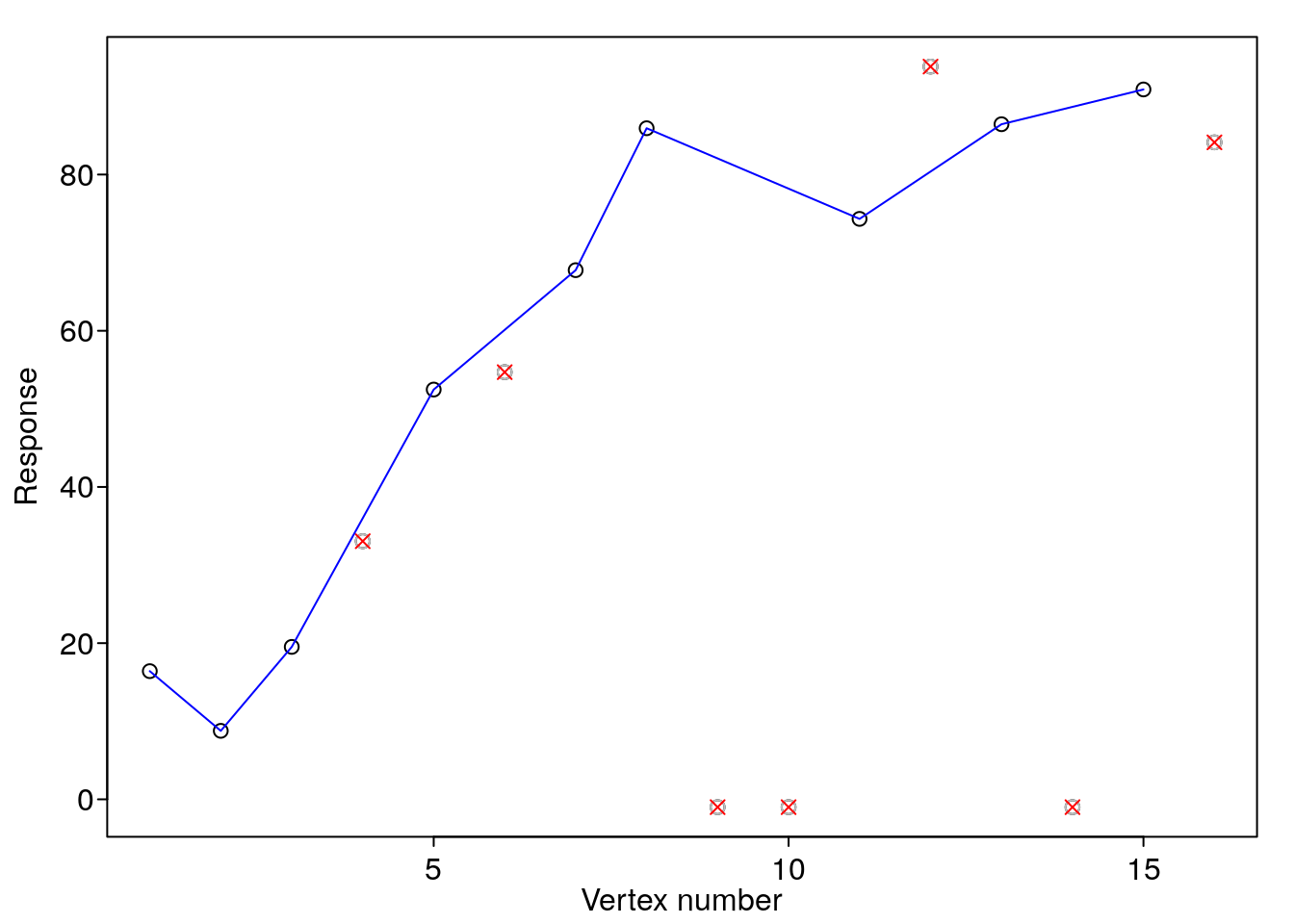Responses vs. vertex number for fixed (right) and a variable (left) step-size simplex optimizationover the response surface `exampleSurfaceR2()`

### Exporting and importing complete simplex

When the experiments take a long time, the information of the optimization can be safely stored in an external file by using the `simplexExport()` function. This function creates a plain text file with a `.smplx` extension that contains all the simplex information. The file must not be edited by hand since it may produce misoperation of some package functions and in the worst case, it can cause the loss of the information

``simplexExport(simplex = simplexR3)``

The `simplexImport()` function imports the simplex information contained into a `.smplx` file. This function should be used when the experimenter is ready to provide responses to the vertexes that do not have it.

``````print(simplexR3, conventions = FALSE)
#> Current simplex:
#>            pH Temp Conc . Response Label Nature
#> Vertex.1: 6.8  330  0.6 |       NA    NA      S
#> Vertex.2: 6.8  350  0.4 |       NA    NA      S
#> Vertex.3: 7.2  350  0.6 |       NA    NA      S
#> Vertex.4: 7.2  330  0.4 |       NA    NA      S
rm(simplexR3)
exists('simplexR3')
#>  FALSE
# We have exported and removed the 'simplexR3' object. Now it will be imported
simplexImport(filename = 'simplexR3')
print(simplexR3, conventions = FALSE)
#> Current simplex:
#>            pH Temp Conc . Response Label Nature
#> Vertex.1: 6.8  330  0.6 |       NA    NA      S
#> Vertex.2: 6.8  350  0.4 |       NA    NA      S
#> Vertex.3: 7.2  350  0.6 |       NA    NA      S
#> Vertex.4: 7.2  330  0.4 |       NA    NA      S``````

## Noisy response surfaces

The response surfaces that describe systems in the real world may significantly differ from that shown in Figure 1. It is impossible to obtain a result without uncertainty when measuring a response in a real system. Those systems may be better represented by noisy response surfaces that are studied in this section. It is vitally necessary to assess the proper variability of the system to determine whether an experiment is better than others in a statistically significant way. A suitable and economic approach to estimate the system variability is to repeat the best and the worst vertexes in the initial simplex (Walters et al. 1991). If the system is too noisy and this noise is not known, the simplex optimization algorithm (and virtually neither any other strategy) may not be capable of improving the process.

The Figure 6 shows different noisy scenarios for the simplex optimization over the response surface `exampleSurfaceR2()`.

``````noises <- c(3, 8, 18)
seeds <- c(0, 13, 13)
for (ii in 1:3) {
prspctv(length = 45, noise = noises[ii], surface = exampleSurfaceR2,
par = list(mar = c(1.2, 1, 0, 0)), ltheta = -120, shade = 0.2, expand = 0.6,
xlab = 'Temperature (K)', ylab = 'pH', zlab = 'Yield (%)', ticktype = "detailed")
set.seed(seeds[ii])
simplexNoisy <- exampleOptimization(surface = exampleSurfaceR2, noise = noises[ii],
centroid = c(7, 340), stepsize = c(1.2, 10))
cntr.ns <- cntr(surface = exampleSurfaceR2, length = 200, noise = noises[ii])
print(addSimplex2Surface(p = cntr(surface = exampleSurfaceR2, length = 200, noise = noises[ii]),
simplex = simplexNoisy))
plotSimplexResponse(simplexNoisy)
}``````